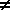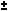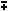# Basic-Algebra-Polynomial-Exponential-Logarithms Identities## Basic Algebra Identities

Closure Property of Addition
Sum (or difference) of 2 reals equals a real number
a + 0 = a
a + (-a) = 0
(a + b) + c = a + (b + c)
a + b = b + a
Definition of Subtractio
a - b = a + (-b)
Closure Property of Multiplication
Product (or quotient if denominator0) of 2 reals equals a real number
Multiplicative Identity
a * 1 = a
Multiplicative Inverse
a * (1/a) = 1   (a0)
(Multiplication times 0)
a * 0 = 0
Associative of Multiplication
(a * b) * c = a * (b * c)
Commutative of Multiplication
a * b = b * a
Distributive Law
a(b + c) = ab + ac
Definition of Division
a / b = a(1/b)## Basic Polynomial Identities

Binomial Theorem
(a+b)2 = a2 + 2ab + b2
Multiply Binomials
(a+b)(c+d) = ac + ad + bc + bd
Difference of Squares
a2 - b2 = (a+b)(a-b)
Sum and Difference of Cubes
a3b3 = (ab)(a2ab + b2)
x2 + (a+b)x + ab = (x + a)(x + b)
If ax2 + bx + c = 0 then x = ( -b(b2 - 4ac) ) / 2a

## Basic Exponential Identities (Powers)

x a x b = x (a + b)
x a y a = (xy) a
(x a) b = x (ab)
x (a/b) = bth root of (x a) = ( bth(x) ) a
x (-a) = 1 / x a
x (a - b) = x a / x b

## Logarithms Basic

y = logb(x) if and only if x=b y
logb(1) = 0
logb(b) = 1
logb(x*y) = logb(x) + logb(y)
logb(x/y) = logb(x) - logb(y)
logb(x n) = n logb(x)
logb(x) = logb(c) * logc(x) = logc(x) / logc(b)##Top Calculators ►

Online Algebra calculation, formulas , Digital calculation, Statistical calculation, Math Converters Pet Age Calculator,